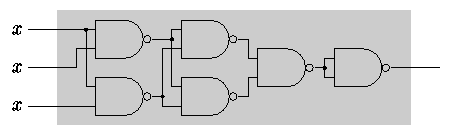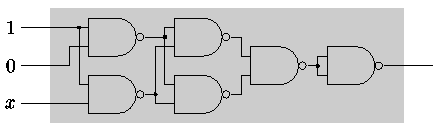Online JudgeProblem SetAuthorsOnline ContestsUser
Web Board
F.A.Qs
Statistical Charts
Problems
Submit Problem
Online Status
Prob.ID:
Register
Authors ranklist
Current Contest
Past Contests
Scheduled Contests
Award Contest
Register
Language:
Gates
 Time Limit: 5000MS Memory Limit: 65536K Total Submissions: 322 Accepted: 144 Special Judge

Description

In contemporary VLSI chip industry, the software tools used by electrical engineers perform many optimizations. Your task is to implement one specific optimization of some chip design. Your tool is given an acyclic net of NAND gates (NAND gate computes the negated conjunction of its inputs, i.e. the output value of the gate is 0 if and only if its both input values are 1). The net is a part of already synthesized component and cannot be changed. All the inputs of the net are connected to one signal x. The objective is to disconnect x from some inputs and to assign constant signals 0 and/or 1 to those inputs in such a way that the function implemented by the design remains unchanged.

We say that an assignment of x's and/or 0's and/or 1's to the inputs of the net is optimal if the number of inputs connected to x is the smallest possible but the net still computes the same function as if all the inputs were connected to x.

Example

Look at the following design.We can change it to the design with only one variable input, for example:(Observe that there are other ways of connecting the inputs to just one x and to some number of 0's and 1's that implement the same function).

Write a program which for each data set:

reads the description of the net,

computes an optimal assignment of x's and/or 0's and/or 1's to the inputs of the net,

writes the result.

Input

The first line of the input contains exactly one positive integer d equal to the number of data sets, 1 <= d <= 20. The data sets follow.

Each data set consists of two consecutive lines. The rst of those lines contains exactly two positive integers n and m separated by single space, 1 <= n <= 100 000, 1 <= m <= 200 000. Integer n is the number of the net inputs and integer m is the number of the gates in the net.

The second of those lines contains exactly 2m nonzero integers, separated by single spaces. The numbers on positions 2j - 1 and 2j describe the signal sources for the inputs to gate j. The positive number s means the output of gate s. The negative number s means the (-s)-th input to the net. The gates and the net inputs are numbered starting from one. The input of each gate is connected to an input of the net or to an output of a gate whose description occurred earlier in the sequence. Each net input is connected to at least one gate input. Each gate output is connected to at least one gate input except the output of the last gate that is connected to the output of the net.

Output

The output should consist of exactly d lines, one line for each data set. The line number i should contain the answer to the i-th data set.

The answer to one data set should consist of a sequence of exactly k characters terminated by the end of line (with no spaces in between). Each of those characters should be 0 (the digit 'zero' ) or 1 (the digit 'one') or x (lower-case letter 'x' ). The i-th symbol of the sequence denotes the assignment to the i-th input of the net.

If there are more than one optimal assignment then your program should output any of them (but only one).

Sample Input

```1
3 6
-1 -3 -1 -2 1 2 1 2 4 3 5 5```

Sample Output

`10x`

Source

[Submit]   [Go Back]   [Status]   [Discuss]Home PageGo BackTo top